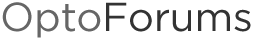# Need To Write A Specific Number of I/O's using Move Numeric Table To I/O Unit Ex

Hello,

I need help with using “Move Numeric Table To I/O Unit Ex” to limit it’s range from writing to all 16 slots of my I/O rack to only the first 14.

I have been using the instruction “Move Numeric Table To I/O Unit Ex” to move every I/O variable from a 512 length (32points*16slots)table to a specific I/O unit for quite some time without any problems. Now starting on slot 15 of a 16 slot Snap rack, I have a PID loop that writes directly to the A/O on channel 0. Since the Move Numeric Table To I/O Unit Ex writes to all the possoble I/O’s on the rack, it will overwrite the PID trying to send an output to slot 15 ch 0. I have tried reducing my 512 length table to 448 but end up with the que error 12 = Invalid table index value—index was negative or greater than or equal to the table size.

Does anyone have any experiance and a workaround for this?

Good question, PilotMan…

As is usually the case, there are several different ways you might approach this problem. A few ideas come to mind. Perhaps a combination or some variation might work for you.

[B]OPTION 1: [/B]
Still use Move Numeric Table to I/O Unit Ex, but with a pointer table rather than a numeric table. That command appears to work fine with a partially loaded table (so if you have nulls after index 448 it doesn’t complain). You just have to keep your non-table write points at the end of the rack, which I believe you said you did. The tricky part is getting that table set up with the values you need. If you’re writing a bunch of identical values it’s not too bad.

[B]OPTION 2:[/B]
You can also write to the individual points in a loop (again, you could have a pointer table, this time pointing to the points), but that wouldn’t do the write as fast as the command you mentioned.

[B]OPTION 3:[/B]
There’s also the SetIoUnitFromMomo command (for digital).

But getting back to that first option I mentioned, let’s look at some sample code. This would write the value of 2.2 to the first 448 points on your rack (32 points per module, 14 modules):

``````// Initialize the data to write into the float called float22
float22 = 2.2;

// Initialize our pointer to a float, so it points to float22
pFloat = &float22;

// Load up the pointer table with that same float pointer for all 448 elements
for my_index = 0 to 447 step 1

my_pointer_table[ my_index ] = &*pFloat;

next

// Write the pointer table (loaded with pointers to that value of 2.2) to I/O Unit called "self"
MoveNumTableToIoUnitEx( my_pointer_table, 0, self, 32 );

``````

I’m guessing you’ll want to set all those points to something other than 2.2. So, using this method, you’d need individual variables for each unique value. For example, let’s pretend we want to assign each point to the value of 1 + it’s point number. We’d need 32 floats, one for each of the 32 points. I could name them float0 to float31 to make it easier to loop through them. Here’s what it would look like for the first three modules on the rack:

``````for my_index = 0 to 31 step 1

// Build the name of the variable, will be float0, float1, float2 ... float31
NumberToString( my_index, sFloatName );
sFloatName = "float" + sFloatName;

// Get a pointer to this float variable so we can use it
// to assign a value to the float variable, and point the table index to it
GetPointerFromName( sFloatName, pFloat );

// Assign index + 1 to the value of what this pointer points to (just as example data)
// So we'll have float0 = 1, float1 = 2 ... float31 = 32
*pFloat = my_index + 1;

// Assign the first three modules on the rack to these sample values,
// in my_pointer_table which will be assigned to the rack when we're done looping
my_pointer_table[ 0 + my_index ] = &*pFloat;
my_pointer_table[ 32 + my_index ] = &*pFloat;
my_pointer_table[ 64 + my_index ] = &*pFloat;

next

// Write the pointer table to I/O Unit called "self"
MoveNumTableToIoUnitEx( my_pointer_table, 0, self, 32 );

``````

I know not everyone is comfortable w/pointers, and the GetPointerFromName command is probably in the realm of “advanced programming,” but it’s not so hard, hopefully examples help!

The most tedious part of this method, especially if you have lots of different values for your points, would be creating all the float variables named float0, float1, … float511. (Which could correspond to a table. Unfortunately, we can’t have one of those pointer table elements point to an individual element in a numeric table at the moment.)

Anyway, did that make sense at all? Clear as mud? Questions? Better suggestions?

-OptoMary